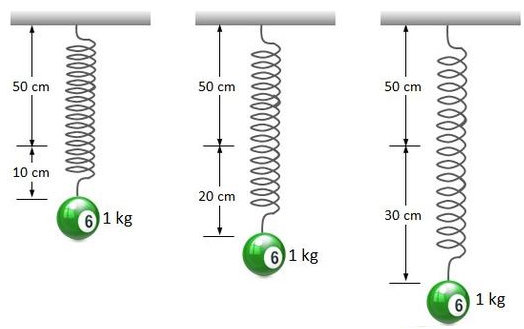# Harmonic springWhen a ball with mass $1\text{ kg}$ is hung on a $50\text{ cm}$ long spring, the length of the spring is increased by $10\text{ cm}$ in steady state, as shown in the far left diagram. Now, suppose that we pull this ball further down and let it go. Then it will show simple harmonic oscillations. What will be the periods of these oscillations when we pull down the ball by $10$ cm and $20$ cm, respectively, as shown in the middle and right diagrams above?

Gravitational acceleration is $10 \text{ m/s}^2$.

×